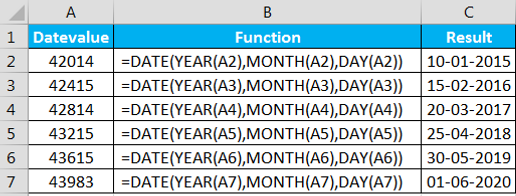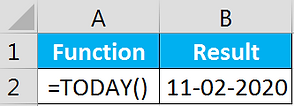# Date & Time Function

Excel has quite a few functions that work with dates. These functions are accessible by choosing Formula >> Function Library >> Date & Time

5.1) Day: The Day function displays the day portion of a date

Syntax = DAY(serial_number)

Example5.2) Date: This function returns a serial date value.

Syntax = DATE(year, month, day)

Example5.3) Month: The Month function displays the month portion of a date.

Syntax = MONTH(serial_number)

Example5.4) Year: The Year function displays the year portion of a date.

Syntax = YEAR (serial_number)

Example5.5) Datevalue: Convert date in number format

Syntax =  DATEVALUE(date_text)

Example5.6) Today: This function display current date.

Syntax = TODAY()

Example5.7) Weekday: This function has two arguments: serial_number and return_type. The serial_number argument is simply a number that represents a date. The return_type argument is optional, and assumed to be 1 if omitted

Syntax = WEEKDAY(serial_number, [return_type])

Example5.8) Timevalue: Returns the decimal number of the time represented by a text string

Syntax = TIMEVALUE(time_text)

Example5.9) Time: Returns the decimal number for a particular time.

Syntax = TIME(hour, minute, second)

Example5.10) Hour: Return the hours portion of a time value

Syntax = HOUR(serial_number)

Example5.11) Second: Return the seconds portion of a time value.

Syntax = SECOND(serial_number)

Example5.12) Minute: Return the minute portion of a time value

Syntax = MINUTE(serial_number)

Example